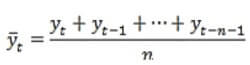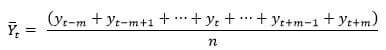# Simple Moving Average Formula

There are two popular methods to calculate Simple moving average. First of all, by presenting a simple definition with a sample, we focus to understand a general concept of 'Simple moving average formula' (See the below video).

The simple moving average computes an average of the last n amounts, where n represents the number of your sample or for which you want the average. For a better clarification, see the first following formula for calculation the Simple moving average:

Simple moving average (watch the video):Simple Moving Average Formula

Where Y refers to the assumed variable, t refers to the cell or position that you want to start from it to get the simple moving average, and n is the 'interval' of simple moving average in your time series, and finally after the summation will done then the summation value divides by n (see the below video).

The second method for calculation of the 'Simple moving average formula' is:Midpoint Moving Average Formula

Where m=Int(n/2), n= the interval of Simple moving average, and t refers to the position of variable (the number of position) that you want to get Simple moving average (see the below video).

The moving average is computed as the summation of N observations, divided by N plotted at the central year in the observation series. Pay attention that in the second Simple moving average formula, N should be an odd number.

In this part, we will review that how to estimate the trend in a set of data by the method of 'Simple moving average formula'? See the excel file in Simple Moving Average Formula

Smoothing of data series is a popular method in different fields of study and sciences, and there are various approaches to implement it. The clearest type of data smoothing is a simple moving average. The simple moving average is a favorite technical index. This formula is also a key belief to engineering and mathematical studies.

Moving averages are often used to help highlight trends. It is frequently applied in statistics, seasonally-adjusted economic and weather forecasting to find out underlying trends. In stock trading, moving average is an index that represents the average value of a security over a given period of time. In business, it's a common practice to calculate a moving average of sales for the last 3 months to define the recent trend.

Let's look at a simple moving average example, as we have the temperatures of a station in the US in F. For better understanding in this sample we put two different 'Simple moving average', namely three-months moving average, and seven- months moving average. Watch the video for learning the concept of 'Simple moving average formula'.

The moving averages can be used to identify the direction of the trend or define potential support and resistance levels. A simple moving average smooths out volatility, and makes it easier to view the variable trend. Simple moving averages are often used to determine trend direction. If the Simple moving average is moving up, the trend is up. If the Simple moving average is moving down, the trend is down.

Name: Hidden